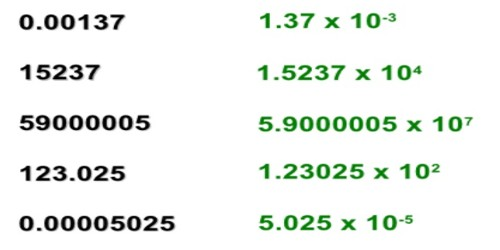Converting Decimal Numbers to Scientific Notation

When working with very large or very small numbers, scientists, mathematicians, and engineers often use scientific notation to express those quantities. Scientific notation uses exponential notation. The following are examples of scientific notation.

Light year: number of miles light travels in one year, about 5,880,000,000,000.

Scientific notation is 5.88 x 1012 miles.

Hydrogen atom: has a diameter of about 0.00000005 mm.

Scientific notation is 5 x 10-8 mm

Scientific notation is used to express very large or very small numbers. A number in scientific notation is written as the product of a number (integer or decimal) and a power of 10. The number has one digit to the left of the decimal point. The power of ten indicates how many places the decimal point was moved.First, factor out one of the powers of ten; either will work, but the smaller one may be easiest. This involves dividing both numbers by the power of ten and multiplying the whole quantity by the same power of ten. To divide one power of ten by another, simply subtract the two exponents (see Multiplication/Division). Next, convert the two numbers from scientific notation to real numbers. Now add the two numbers normally. Finally convert to scientific notation if the coefficient is less than 1 or greater than 10.

The decimal number 0.00000065 written in scientific notation would be 6.5×10-7 because the decimal point was moved 7 places to the right to form the number 6.5. It is equivalent to 6.5*0.1*0.1*0.1*0.1*0.1*0.1*0.1.

When the number is 10 or greater, the decimal point has to move to the left, and the power of 10 is positive. When the number is smaller than 1, the decimal point has to move to the right, so the power of 10 is negative.

Example: 0.0055 is written 5.5 × 10-3

Because 0.0055 = 5.5 × 0.001 = 5.5 × 10-3

A decimal number smaller than 1 can be converted to scientific notation by decreasing the power of ten by one for each place the decimal point is moved to the right.

Scientific notation numbers may be written in different forms. The number 6.5×10-7 could also be written as 6.5e-7.

Information Source: